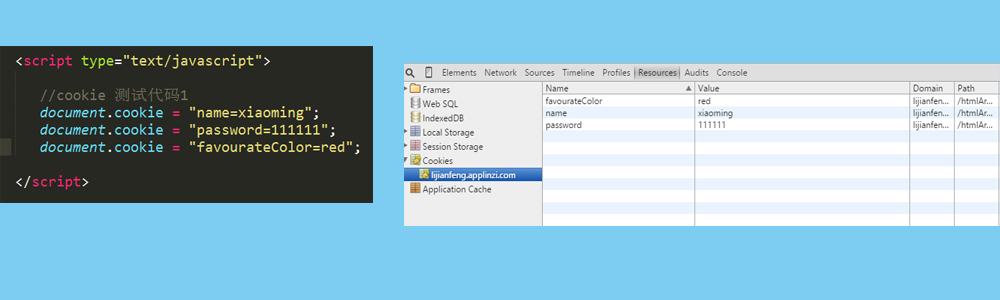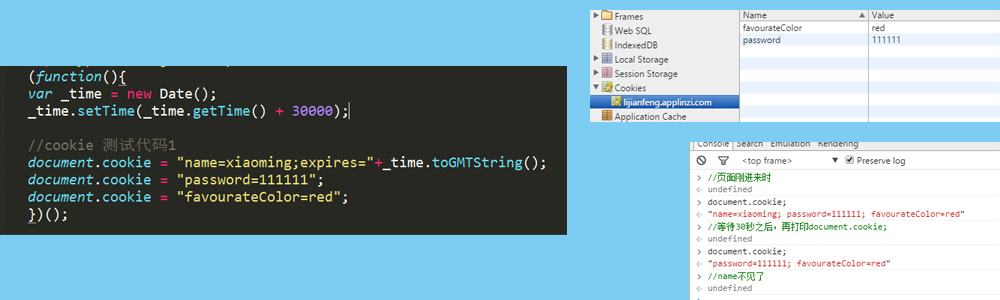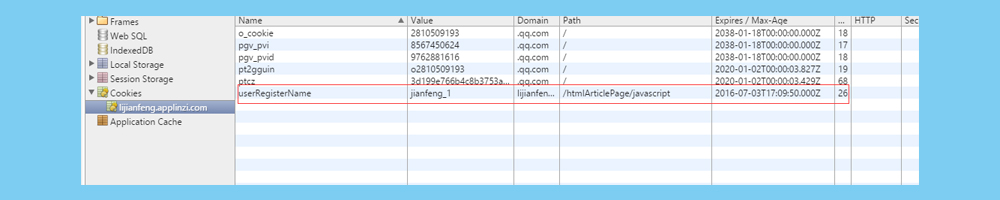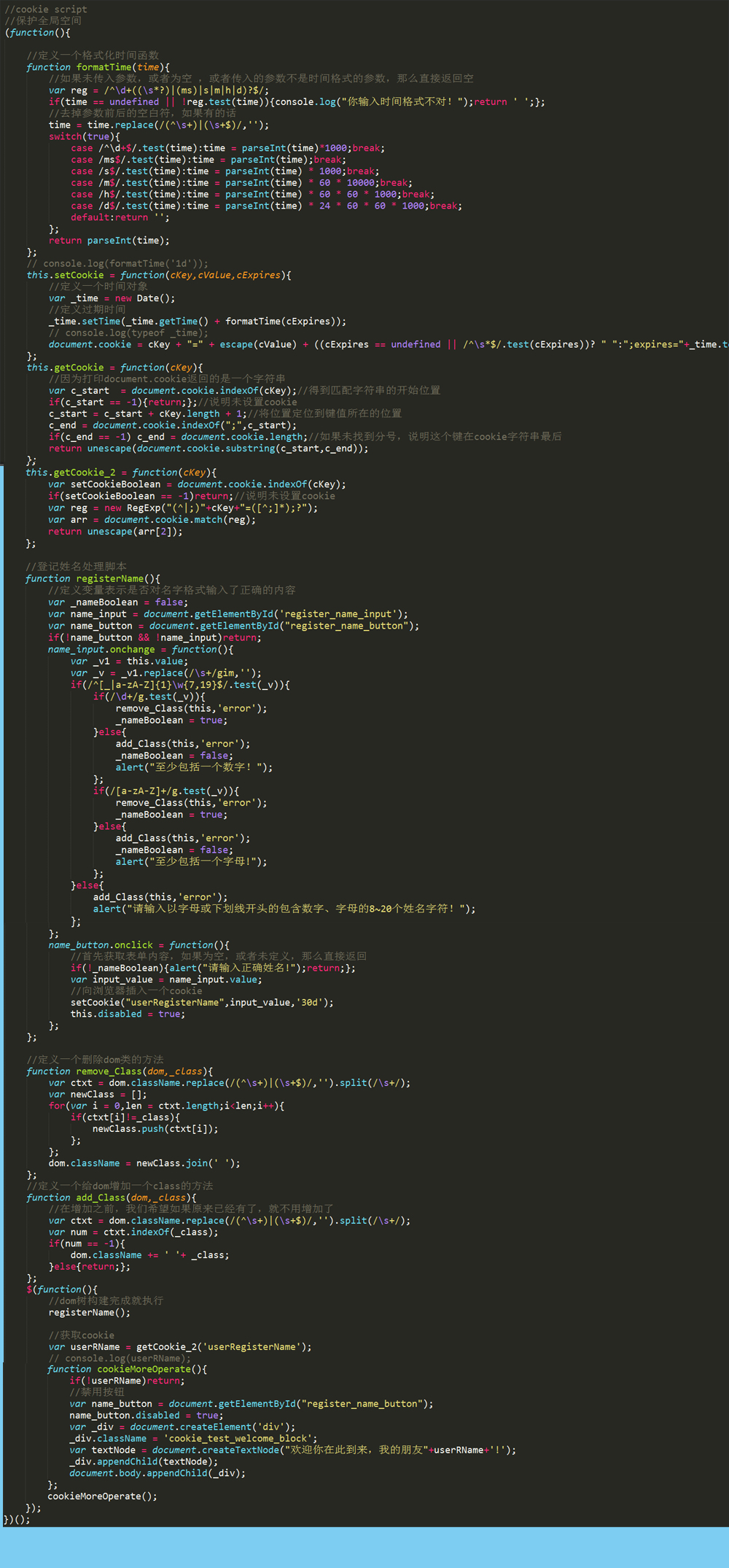## 基础介绍

``` document.cookie = "name=xiaoming"; document.cookie = "password=111111"; document.cookie = "favourateColor=red"; `````` var _time = new Date();//实例化一个时间对象 _time = _time.setTime(_time.getTime() + 30000);//30秒之后过期 document.cookie = "name=xiaoming;expires="+_time.toGMTString(); `````` _time = _time.setTime(_time.getTime() - 1000); ```

``` document.cookie = "name1=xiaochen;path=/htmlArticlePage/css/"; ```

``` document.cookie = "name1=xiaochen;domain=t1.applinzi.com;path=/"; ```

## 实战

### 需求

``` this.setCookie = function(cKey,cValue,cExpires){ //定义一个时间对象 var _time = new Date(); //定义过期时间 _time.setTime(_time.getTime() + formatTime(cExpires)); document.cookie = cKey + "=" + escape(cValue) + ((cExpires == undefined || /^\s*\$/.test(cExpires))? " ":";expires="+_time.toGMTString()); }; ```

``` //定义一个格式化时间函数 function formatTime(time){ //如果未传入参数，或者为空 ，或者传入的参数不是时间格式的参数，那么直接返回空 var reg = /^\d+((\s*?)|(ms)|s|m|h|d)?\$/; if(time == undefined || !reg.test(time)){console.log("你输入时间格式不对！");return ' ';}; //去掉参数前后的空白符，如果有的话 time = time.replace(/(^\s+)|(\s+\$)/,''); switch(true){ case /^\d+\$/.test(time):time = parseInt(time)*1000;break; case /ms\$/.test(time):time = parseInt(time);break; case /s\$/.test(time):time = parseInt(time) * 1000;break; case /m\$/.test(time):time = parseInt(time) * 60 * 10000;break; case /h\$/.test(time):time = parseInt(time) * 60 * 60 * 1000;break; case /d\$/.test(time):time = parseInt(time) * 24 * 60 * 60 * 1000;break; default:return ''; }; return parseInt(time); }; ```

``` name_input.onchange = function(){ var _v1 = this.value; var _v = _v1.replace(/\s+/gim,''); if(/^[_|a-zA-Z]{1}\w{7,19}\$/.test(_v)){ if(/\d+/g.test(_v)){ remove_Class(this,'error'); }else{ add_Class(this,'error'); alert("至少包括一个数字！"); }; if(/[a-zA-Z]+/g.test(_v)){ remove_Class(this,'error'); }else{ add_Class(this,'error'); alert("至少包括一个字母!"); }; }else{ add_Class(this,'error'); alert("请输入以字母或下划线开头的包含数字、字母的8~20个姓名字符！"); }; }; ```

``` //定义一个删除dom类的方法 function remove_Class(dom,_class){ var ctxt = dom.className.replace(/(^\s+)|(\s+\$)/,'').split(/\s+/); var newClass = []; for(var i = 0,len = ctxt.length;i<len;i++){ if(ctxt[i]!=_class){ newClass.push(ctxt[i]); }; }; dom.className = newClass.join(' '); }; //定义一个给dom增加一个class的方法 function add_Class(dom,_class){ //在增加之前，我们希望如果原来已经有了，就不用增加了 var ctxt = dom.className.replace(/(^\s+)|(\s+\$)/,'').split(/\s+/); var num = ctxt.indexOf(_class); if(num == -1){ dom.className += ' '+ _class; }else{return;}; }; ```

``` name_button.onclick = function(){ //首先获取表单内容，如果为空，或者未定义，那么直接返回 if(!_nameBoolean){alert("请输入正确姓名!");return;}; var input_value = name_input.value; //向浏览器插入一个cookie setCookie("userRegisterName",input_value,'30d'); this.disabled = true; }; `````` this.getCookie = function(cKey){ //因为打印document.cookie返回的是一个字符串 var c_start = document.cookie.indexOf(cKey);//得到匹配字符串的开始位置 if(c_start == -1){return;};//说明未设置cookie c_start = c_start + cKey.length + 1;//将位置定位到键值所在的位置 c_end = document.cookie.indexOf(";",c_start); if(c_end == -1) c_end = document.cookie.length;//如果未找到分号，说明这个键在cookie字符串最后 return unescape(document.cookie.substring(c_start,c_end)); }; ```

``` this.getCookie_2 = function(cKey){ var setCookieBoolean = document.cookie.indexOf(cKey); if(setCookieBoolean == -1)return;//说明未设置cookie var reg = new RegExp("(^|;)"+cKey+"=([^;]*);?"); var arr = document.cookie.match(reg); return unescape(arr); }; ```

``` //获取cookie var userRName = getCookie_2('userRegisterName'); console.log(userRName); `````` function cookieMoreOperate(){ if(!userRName)return; //禁用按钮 var name_button = document.getElementById("register_name_button"); name_button.disabled = true; }; cookieMoreOperate(); ```

``` function cookieMoreOperate(){ if(!userRName)return; //禁用按钮 var name_button = document.getElementById("register_name_button"); name_button.disabled = true; var _div = document.createElement('div'); _div.className = 'cookie_test_welcome_block'; var textNode = document.createTextNode("欢迎你在此到来，我的朋友"+userRName+'!'); _div.appendChild(textNode); document.body.appendChild(_div); }; cookieMoreOperate(); ```